# Conjugate equation

Find the numbers of a and b, if (a - bi) (3 + 5i) is the Conjugate of (-6 - 24i)

a =  3
b =  -3

### Step-by-step explanation: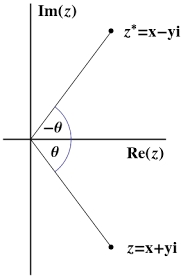Did you find an error or inaccuracy? Feel free to write us. Thank you!Tips to related online calculators
Do you have a system of equations and looking for calculator system of linear equations?
Try our complex numbers calculator.

## Related math problems and questions:

• Linear imaginary equationGiven that 2(z+i)=i(z+i) "this is z star" Find the value of the complex number z.
• Imaginary numbersFind two imaginary numbers whose sum is a real number. How are the two imaginary numbers related? What is its sum?
• Eq2 equationsFor each of the following problems, determine the roots of the equation. Given the roots, sketch the graph and explain how your sketch matches the roots given and the form of the equation: g(x)=36x2-12x+5 h(x)=x2-4x+20 f(x)=4x2-24x+45 p(x)=9x2-36x+40
• ABS CNCalculate the absolute value of complex number -15-29i.
• Find d 2Find d in an A. P. whose 5th term is 18 and 39th term is 120.
• Two grandmothersTwo grandmothers went to sell eggs at the market, and they had a total of 100. When they sold all the eggs, they made the same money. The first grandmother says to the second: "If I sold my eggs for your price, I would earn 15 crowns. " The other grandmot
• Mappings of complex numbers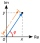Find the images of the following points under mappings: z=3-2j w=2zj+j-1
• Three numbers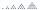What are three numbers that have the property: the sum of the first and second numbers' reciprocals is 12/7, the first and third 11/24, and the second and the third 3/8.
• Two math problems1) The sum of twice a number and -6 is nine more than the opposite of that number. Find the number. 2) A collection of 27 coins, all nickels, and dimes, is worth \$2.10. How many of each coin are there? The dime, in United States usage, is a ten-cent coin.
• Difference of two numberThe difference between the two numbers is 20. They are positive integers greater than zero. The first number raised to one-half equals the second number. Determine the two numbers.
• School year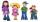At the end of the school year has awarded 20% of the 250 children who attend school. Award got 18% boys and 23% of girls. Find how many boys and how many girls attend school.
• The modulus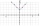Find the modulus of the complex number 2 + 5i
• Distance two imaginary numbsFind the distance between two complex number: z1=(-8+i) and z2=(-1+i).
• Find the 21Find the sum of the six terms of the finite geometric sequence 96, -48, 24, -12
• Odd numbersThe sum of four consecutive odd numbers is 1048. Find those numbers ...
• Intersections 3Find the intersections of the circles x2 + y2 + 6 x - 10 y + 9 = 0 and x2 + y2 + 18 x + 4 y + 21 = 0
• Ratio three numbers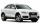Three numbers SUV are in the ratio 1:2:3. Their sum is 24. Find this numbers and write their add and sum.